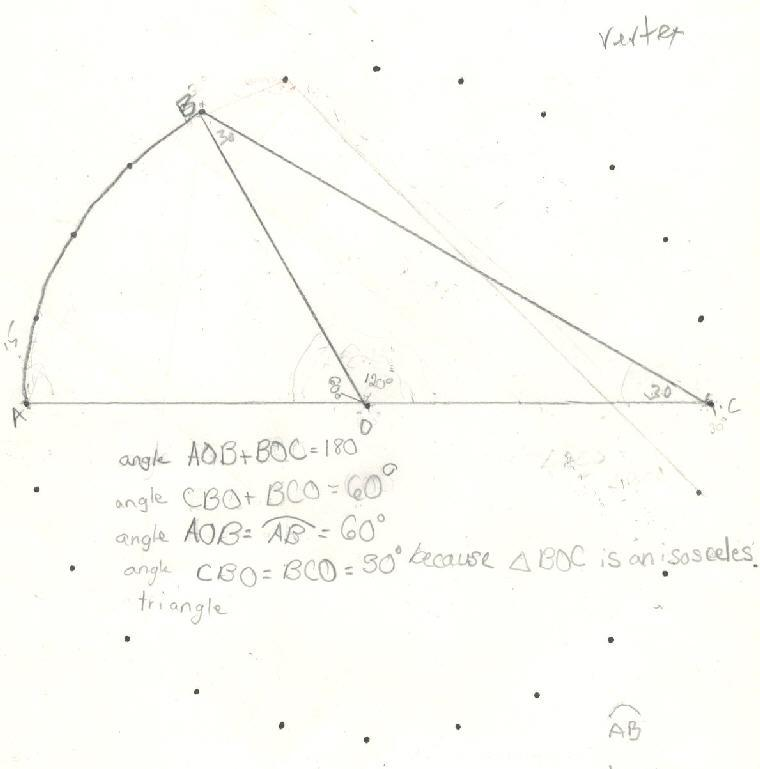Sheri, a 5th grader, finds the measure of an inscribed angle

Sheri wrote a geometric proof, in her own way to show the inscribed angle is 30', when its arc is 60'. Fine job Sheri!Sheri writes 64 using exponents
Sheri solves the quadratic equation x2 - x - 1 = 0
Sheri finds the base for Don's age of   114? = 7110
Sheri uses the quadratic formula to find base for Don's age of   114? = 7110
Sheri uses binary numerals to make the Magic Number Game cards
Sheri changes the shape of a dog using matrices
Sheri enlarges a shell using the pantograph
Sheri moves a parabola and finds the equation
Sheri finds the ratio of The Volume of a Pyramid / The Volume of a Cube (3 ways)
Sheri works with the sand pendulum
Sheri figures out a rule for The Tower Puzzle
Sheri starts Trig

To other discoveries

To order Don's materials
Mathman home